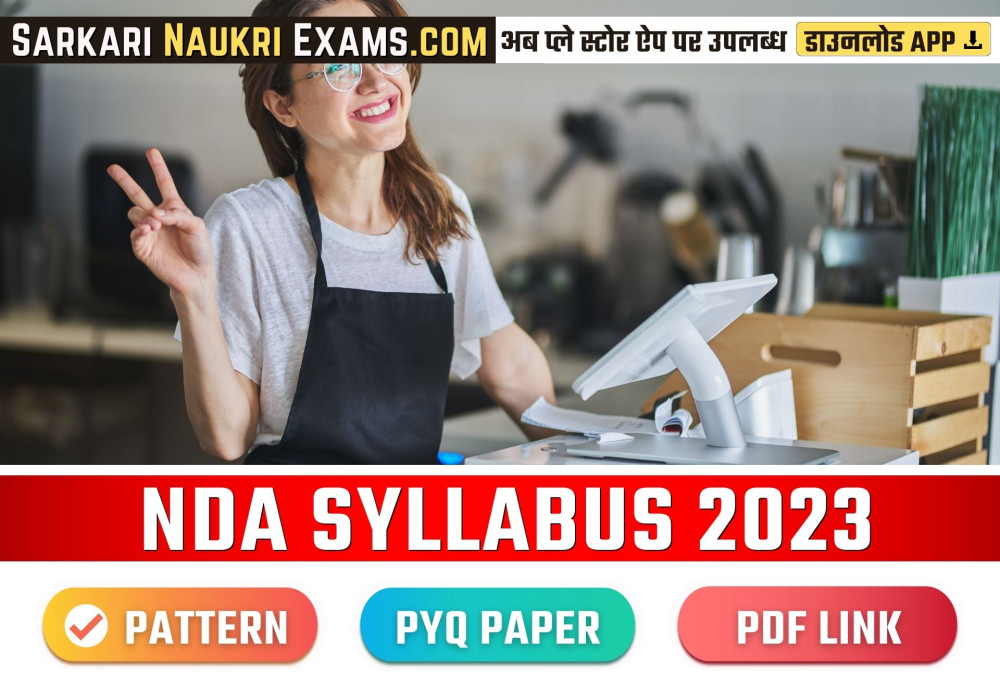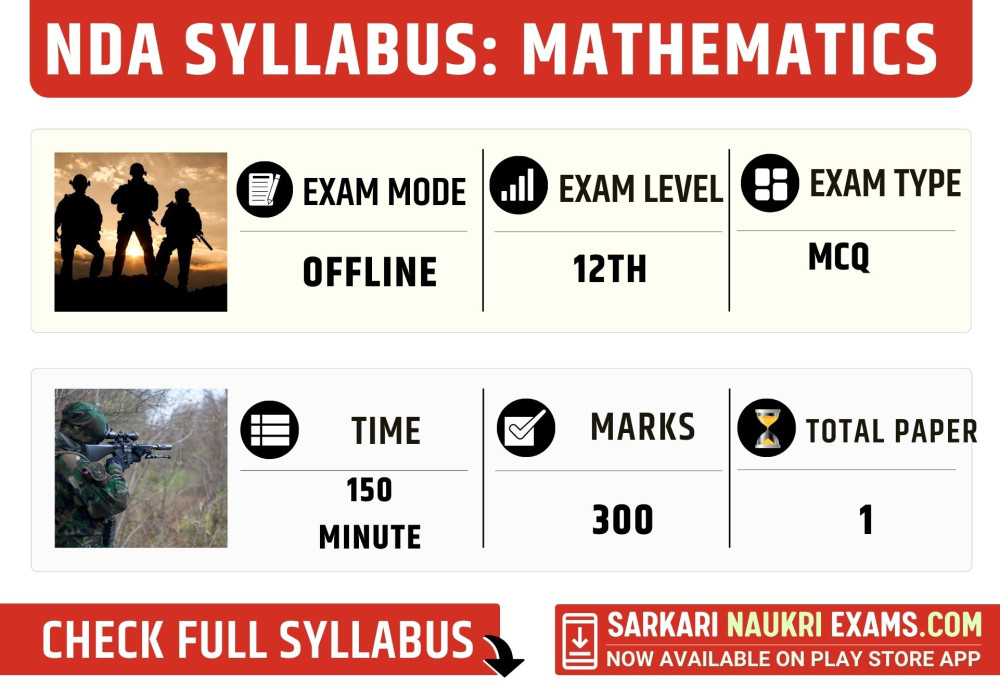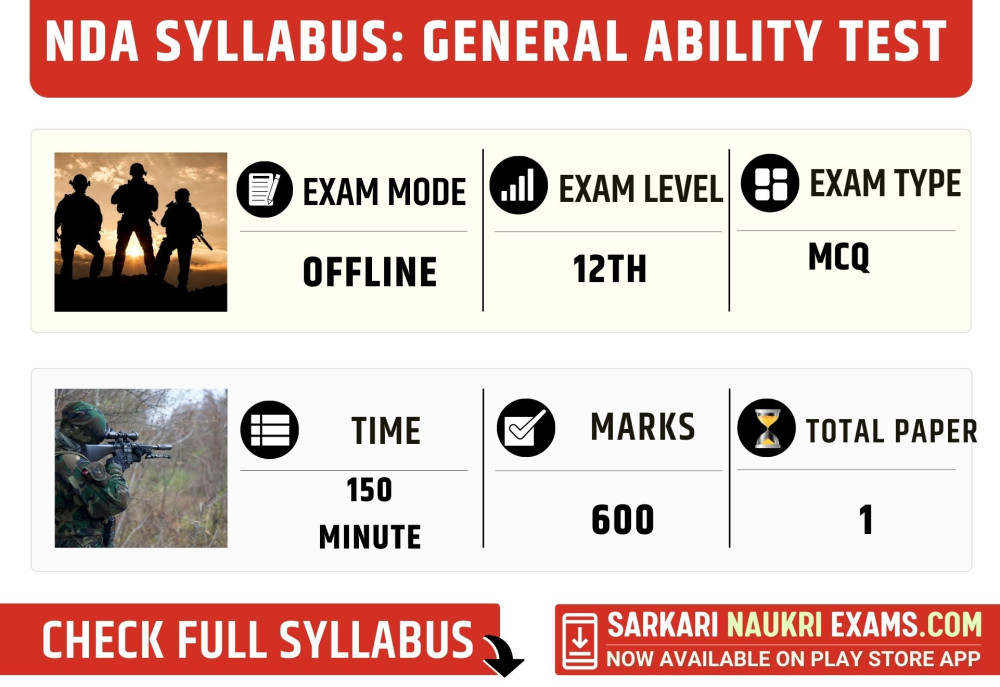The Union Public Service Commission conducts the NDA examination, which is a prestigious examination for those who aspire to join the Indian Armed Forces. The NDA syllabus PDF is available on the official website of UPSC. The syllabus includes both Mathematics and General Ability Tests. The NDA syllabus for girls is the same as that for boys. The NDA syllabus 2023 PDF is also available on the website. The NDA syllabus in Hindi is also available for the convenience of Hindi-speaking candidates. The NDA syllabus 2023 for girls is also the same as that for boys.

The Mathematics section of the exam consists of 120 questions and carries 300 marks, while the General Ability Test consists of 150 questions and carries 600 marks, making a total of 270 questions and 900 marks. As per the NDA Exam Pattern 2023, the exam is of five hours duration and is objective-type. Candidates should be well-prepared for both Mathematics and General Ability Test sections to ace the NDA exam.

### NDA Syllabus 2023: Paper-I MathematicsThe NDA examination conducted by the Union Public Service Commission includes various subjects, one of which is Mathematics. The Mathematics paper is conducted in offline mode using OMR sheets and is of an intermediate level, with objective-type questions in the form of multiple-choice questions. The paper is available in both Hindi and English, except for the language subjects.

The total number of questions in the Mathematics paper is 120, with a total of 300 marks. The total time allotted for the examination is 150 minutes. For each correct answer, the candidate is awarded 2.5 marks, while for each incorrect answer, 0.83 marks are deducted as negative marking. The Mathematics paper comprises questions related to General Mathematics. The cut-off marks for the Mathematics paper are decided by the UPSC.

Organization

The Union Public Service Commission

Exam Mode

Offline (OMR)

Exam Level

12th (Intermediate)

Exam Type

Objective (MCQ)

Paper Medium

Hindi & English (Except language subject)

Total Question

120

Total Marks

300

Total Time (Minutes)

150

2.5

Negative Marking

0.83

Cut Off Marks

Paper

Paper 1

Subject Details

Name Qsn Marks
General Mathematics 120 300

1. 📖 General Mathematics

Total Qsn 120 Total Marks- 300 Total Time- 120(Mins)

Algebra:

1. Sets, Venn diagrams, De Morgan laws, Cartesian product, relation, equivalence relation.
2. Real numbers, Complex numbers, Modulus, Cube roots, Conversion of a number in Binary system to Decimals, and vice-versa.
3. Arithmetic, Geometric and Harmonic progressions.
4. Quadratic equations, Linear inequations, Permutation and Combination, Binomial theorem, and Logarithms.

Calculus:

1. Concept of a real-valued function, domain, range, and graph of a function.
2. Composite functions, one-to-one, onto, and inverse functions.
3. The notion of limit, Standard limits, Continuity of functions, algebraic operations on continuous functions.
4. Derivative of function at a point, geometrical and physical interpretation of a derivative-application.
5. Derivatives of sum, product, and quotient of functions, a derivative of a function concerning another function, the derivative of a composite function.
6. Second-order derivatives.
7. Increasing and decreasing functions.
8. Application of derivatives in problems of maxima and minima.

Matrices and Determinants:

1. Types of matrices, operations on matrices.
2. Determinant of a matrix, basic properties of determinants.
3. Adjoint and inverse of a square matrix, Applications-Solution of a system of linear equations in two or three unknowns by Cramer’s rule and by Matrix Method.

Integral Calculus and Differential Equations:

1. Integration as inverse of differentiation, integration by substitution and by parts, standard integrals involving algebraic expressions, trigonometric, exponential, and hyperbolic functions.
2. Evaluation of definite integrals—determination of areas of plane regions bounded by curves—applications.
3. Definition of order and degree of a differential equation, formation of a differential equation by examples.
4. General and particular solution of differential equations, solution of the first order, and first-degree differential equations of various types—examples.
5. Application in problems of growth and decay.

Trigonometry:

1. Angles and their measures in degrees and radians.
2. Trigonometric ratios. Trigonometric identities Sum and difference formulae.
3. Multiple and Sub-multiple angles. Inverse trigonometric functions.
4. Applications-Height and distance, properties of triangles.

Vector Algebra:

1. Vectors in two and three dimensions, magnitude, and direction of a vector.
2. Unit and null vectors, the addition of vectors, scalar multiplication of a vector, scalar product, or dot product of two vectors.
3. Vector product or cross product of two vectors.
4. Applications—work done by a force and moment of a force and in geometrical problems.

Analytical Geometry Of Two and Three Dimension:

1. Rectangular Cartesian Coordinate system. Distance formula.
2. Equation of a line in various forms. The angle between two lines.
3. Distance of a point from a line.
4. Equation of a circle in standard and a general form.
5. Standard forms of parabola, ellipse, and hyperbola.
6. Eccentricity and axis of a conic.
7. Point in a three-dimensional space, the distance between two points.
8. Direction Cosines and direction ratios. Equation two points.
9. Direction Cosines and direction ratios. Equation of a plane and a line in various forms.
10. The angle between two lines and the angle between two planes.
11. Equation of a sphere.

Statistics and Probability:

1. Probability: Random experiment, outcomes, and associated sample space, events, mutually exclusive and exhaustive events, impossible and certain events.
2. Union and Intersection of events. Complementary, elementary, and composite events.
3. Definition of probability—class

### NDA Syllabus 2023:Paper -II General Ability TestThe NDA examination conducted by the Union Public Service Commission includes a General Ability Test (Paper II), which is an important subject that tests the candidate's overall knowledge and aptitude. The General Ability Test paper is conducted offline using OMR sheets and is of an intermediate level, with objective-type questions in the form of multiple-choice questions. The paper is available in both Hindi and English, except for the language subjects. The total number of questions in the General Ability Test paper is 150, with a total of 600 marks. The total time allotted for the examination is 150 minutes. For each correct answer, the candidate is awarded four marks, while for each incorrect answer, 1/4th marks are deducted as negative marking.

The General Ability Test paper comprises two subjects: General Knowledge and General English. The General Knowledge section comprises 100 questions with a total of 400 marks, while the General English section comprises 50 questions with a total of 200 marks. The cut-off marks for the General Ability Test paper are decided by the UPSC.

Organization

The Union Public Service Commission

Exam Mode

Offline (OMR)

Exam Level

12th (Intermediate)

Exam Type

Objective (MCQ)

Paper Medium

Hindi & English (Except language subject)

Total Question

150

Total Marks

600

Total Time (Minutes)

150

04

Negative Marking

-1/4

Cut Off Marks

Paper

Paper 1

Subject Details

Name Qsn Marks
General Knowledge 100 400
General English 50 200

1. 📖General Knowledge

Total Qsn 100 Total Marks- 400
• Polity
• Economy
• History
• Geography
• Current Affairs
• Art and Culture
• Science and Technology

2. 📖 General English

Total Qsn 50 Total Marks- 200
1. Spotting Errors:
• Involves identifying mistakes in grammar, punctuation, spelling, and syntax in a sentence or passage.
• Requires knowledge of the rules of the language, including verb tenses, subject-verb agreement, pronoun usage, and more.
• Helps improve writing skills and avoid common errors.
1. Comprehension:
• Refers to the ability to understand written or spoken language.
• Involves identifying the main idea, supporting details, and tone of a passage or conversation.
• Requires strong reading and listening skills, as well as the ability to infer meaning from context.
1. Selecting Words:
• Involves choosing the appropriate word for a given context or sentence.
• Requires knowledge of vocabulary, grammar, and idiomatic expressions.
• Helps improve communication skills and avoid using incorrect or inappropriate words.
1. Ordering of Words in a Sentence:
• Involves arranging words in a grammatically correct and meaningful order in a sentence.
• Requires an understanding of sentence structure, syntax, and word order.
• Helps improve writing and speaking skills and make communication more effective.
1. Sentence Improvement:
• Involves identifying errors or weaknesses in a sentence and improving its clarity, coherence, and correctness.
• Requires knowledge of grammar, syntax, and vocabulary.
• Helps improve writing and communication skills and make the message more effective.
1. Antonyms:
• Refers to words that have opposite meanings.
• Requires knowledge of vocabulary and an understanding of context.
• Helps improve reading and writing skills and expand vocabulary.
1. Synonyms:
• Refers to words that have similar or identical meanings.
• Requires knowledge of vocabulary and an understanding of context.
• Helps improve reading and writing skills and expand vocabulary.

### NDA Syllabus 2023: InterviewDuring the NDA - SSB Interview, candidates are evaluated on various aspects of their personality and skills. The interview process is divided into two stages, with multiple tests in each stage.

In the first stage, candidates undergo the Screening Test, which includes a verbal and non-verbal test and the Picture Perception and Discussion Test (PPDT). Additionally, there are three psychological tests, namely the Thematic Apperception Test (TAT), Word Association Test (WAT), and Situation Reaction Test (SRT). The Self Description Test (SD) is also a part of this stage, along with group discussions (GD), group planning exercises (GPE), and progressive group tasks (PGT).

Candidates who clear the first stage then proceed to the second stage, which comprises the Group Testing Officers (GTO) Test. The GTO Test includes a series of activities that assess the candidate's ability to work in a group, including the Half Group Task (HGT), Individual Obstacle Task (1OT), Command Task, Snake Race/Group Obstacle Race, and the Final Group Task (FGT).

Finally, candidates undergo a Personal Interview and Conference with the board members. This interview assesses the candidate's confidence, communication skills, and suitability for a career in the armed forces.

Here's a table showcasing the NDA - SSB Interview Pattern:

Stage Tests Included
Stage 1 Screening Test
Stage 2 Psychological Test, Group Testing Officers Test
Personal Interview & Conference -

The above table presents the various tests included in the NDA - SSB Interview, categorized according to the stage in which they are conducted.### NDA Syllabus 2023 FAQs

⭐The NDA examination is conducted by whom, and what does it entail?

The NDA examination is conducted by the Union Public Service Commission (UPSC) to select candidates for the National Defence Academy.

⭐What does the NDA examination pattern consist of?

The NDA examination includes two papers: Mathematics (Paper I) and General Ability Test (Paper II). Both papers are conducted offline using OMR sheets.

⭐What is the level of difficulty of the NDA examination?

The NDA examination is of an intermediate level, which is equivalent to the 12th standard.

⭐What is the structure of the questions in the NDA examination?

The NDA examination questions are in the form of multiple-choice questions (MCQs), which are objective in nature.

⭐What is the medium of the NDA examination?

The NDA examination is available in both Hindi and English, except for the language subjects

#### Comment

×

S

Sarkari Naukri Exams-

Thanks for visiting us!

We will reply within 24 Hours.

Thanks & Regards!

Sarkari Naukri Exams.

Updated: